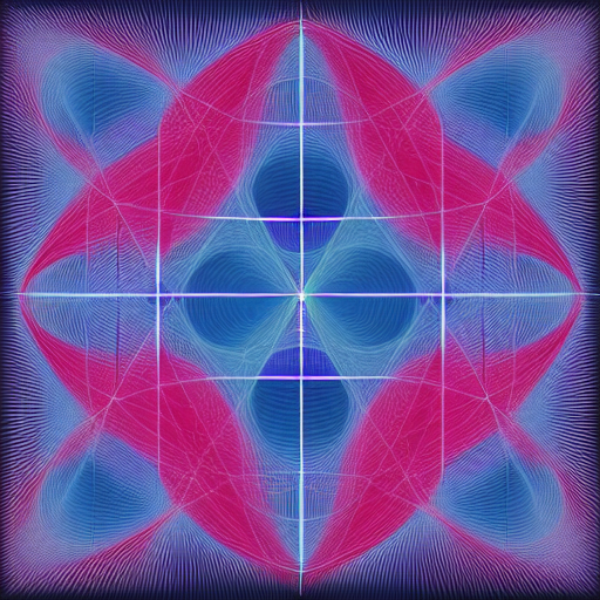Supervised learning experiments involving real quadratic fields lead to machine-learned formulas for class numbers 1, 2 and 3, for our dataset.

## Machine learning class numbers of real quadratic fields

Int J Data Sci Math Sci (2023)

M. Amir, Y. He, K. Lee, T. Oliver, E. Sultanow

We implement and interpret various supervised learning experiments involving real quadratic fields with class numbers 1, 2 and 3. We quantify the relative difficulties in separating class numbers of matching/different parity from a data-scientific perspective, apply the methodology of feature analysis and principal component analysis, and use symbolic classification to develop machine-learned formulas for class numbers 1, 2 and 3 that apply to our dataset.

Int J Data Sci Math Sci (2023)

M. Amir, Y. He, K. Lee, T. Oliver, E. Sultanow